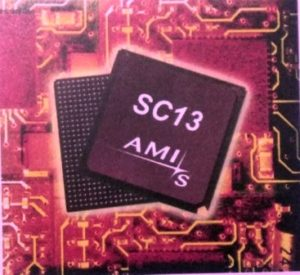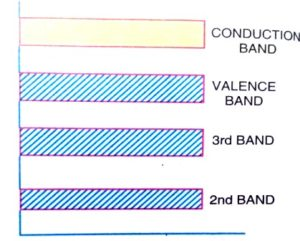# What is semiconductor? Properties and Types of semiconductor

## WHAT IS  SEMICONDUCTOR?

A semiconductor is defined as a substance whose electrical conductivity is intermediate between metallic conductors and non-metallic insulators.

In other words, we can also define semiconductor as the substance which has resistivity (10-4 to 0.5 Ωm) in between metallic conductors and non-metallic insulators. Examples of semiconductors are germanium, silicon, selenium, etc.

### Properties of Semiconductor

• Its resistivity is less than an insulator but more than a conductor.
• Its conductivity is more than an insulator but less than a conductor.
• It has a negative resistance temperature coefficient, which means that an increase in temperature resistance of semiconductors will decrease or vice-versa.
• Current conducting properties will be significantly changed when a metallic impurity (eg. arsenic, gallium, antimony, etc.) is added to it.### Effect of Temperature on semiconductor

Due to the variation in the temperature, the electrical conductivity of a semiconductor changes significantly. Now we will see how at absolute zero and above absolute zero temperature its properties changes.

All the electrons are tightly held by the atoms of the semiconductor at absolute zero temperature. Here inner orbit electrons are bounded whereas the valence electrons are engaged in covalent bonding.

The covalent bonds are very strong and there are no free electrons at absolute zero temperature. Therefore its crystal behaves as a perfect insulator.

In terms of energy bands, the valence band is filled and there is a large energy gap between the valence band and the conduction band. So no electrons from the valence band can reach the conduction band to become a free electron. This non-availability of free electrons makes it behaves like an insulator.At above absolute zero temperature, when the temperature of the semiconductor is raised some of the covalent bonds in the semiconductor break due to the thermal energy given.

When the covalent bond breaks then the electrons engaged in the covalent bonds get free. And a very small amount of electric current is constituted by these free electrons if a potential difference is applied across the semiconductor crystal.In terms of energy band, when the temperature is raised some of the valence band electrons get sufficient energy and moves from the valence band to the conduction band, and thus become free electrons.

And under the influence of an electric field, these free electrons will constitute an electric current.### Types of Semiconductor

On the basis of purity, semiconductors are classified as Intrinsic semiconductors and Extrinsic semiconductors.

#### Intrinsic semiconductor

An intrinsic semiconductor is a pure semiconductor material in which no impurity has been added deliberately.

In the case of an intrinsic semiconductor, hole-electron pairs are created even at room temperature. When the electric field is applied across an intrinsic semiconductor then the conduction of current takes place by processes, i.e by free electrons and holes.

The free electrons are produced due to the breaking up of some covalent bonds by thermal energy. At the same time, holes are also created in the covalent bonds. Under the effect of the electric field conduction through the semiconductor is done by both free electrons and holes.

Therefore the total current inside the semiconductor is the sum of currents due to free electrons and holes.

The crystalline form of silicon(si) and Germanium(Ge) are examples of intrinsic semiconductors. The crystal structure of silicon or germanium is quite similar to that of carbon in a diamond.

The four valence electrons form four covalent bonds with the neighboring atoms. Each bond has two electrons, one from each atom. Each atom is thus surrounded by a completely filled and stable shell of 8 electrons.

At low-temperature say, 0k, all valence electrons in the crystal are bound so strongly that no free electrons are available to conduct current through germanium. Hence a pure germanium crystal at 0k is practically a non-conductor of electricity.

The minimum energy to break a covalent bond is 1.21eV for Si and 0.785Evfor Ge. At room temperature, thermal vibrations of the atom may break up some of the bonds. Thus some electrons may be released which are free to move throughout the crystal.

When an electron breaks away from the covalent bond, the empty place or the valence space left behind in the crystal structure is called a hole.

The motion of an electron that is bounded to another electron is equivalent to the motion of the hole in the opposite direction. Hence hole may be regarded as a particle similar to an electron, but having a positive and equal charge.

#### Extrinsic Semiconductor

A pure intrinsic semiconductor is of no practical significance as it has little current conduction capability at room temperature.

So to increase its electrical conductivity and make it suitable for practical use a small amount of suitable impurity is added to the intrinsic semiconductor then the resulting material is called an impure or extrinsic semiconductor. And the process of adding impurities to a semiconductor is known as doping.

The impurities added to it are either a pentavalent impurity i.e having 5 valence electrons or a trivalent impurity i.e having 3 valence electrons.

When a pentavalent impurity is added to it then a large number of free electrons are produced in it. On the other hand when a trivalent impurity is added to a semiconductor then it creates a large number of holes in it.

Depending upon the type of impurity added, extrinsic semiconductors are divided into two categories,

1.N-type or Donor type semiconductor

2.P-type or Acceptor type semiconductor

 N-type semiconductor P-type semiconductor A type of semiconductor in which large number (surplus) of electrons are present is known as n-type semiconductor. A type of semiconductor in which large number (surplus)of holes are present is known as p-type semiconductor. Electrons are majority charge carriers. Holes are majority charge carriers. Holes are minority charge carriers. Electrons are minority charge carriers. Number of free electrons are greater than holes. Number of holes are greater than free electrons. To obtain n-type semiconductor pentavalent elements (Arsenic, Antimony, or Phosphorus) are used for doping with the semiconductor materials. To obtain p-type semiconductor trivalent elements (Gallium, Boron, or Indium) are used for doping with the semiconductor materials.

I hope you like the article on what is semiconductor? For more articles related to electrical engineering click on the link given below.

VIEW MORE…